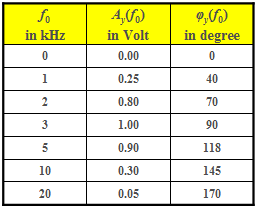# Exercise 1.2Z: Measurement of the Frequency ResponseMeasured signal amplitudes
and phases for filter  $\rm B$

For the metrological determination of the filter frequency response a sinusoidal input signal with an amplitude of  $2 \hspace{0.05cm} \text{V}$  and given frequency  $f_0$  is applied.  The output signal  $y(t)$  or its spectrum  $Y(f)$  are then determined according to magnitude and phase.

• The magnitude spectrum at the output of filter  $\rm A$  with frequency  $f_0 = 1 \ \text{kHz}$  is:
$$|Y_{\rm A} (f)| = 1.6\hspace{0.05cm}{\rm V} \cdot {\rm \delta } (f \pm f_0) + 0.4\hspace{0.05cm}{\rm V} \cdot {\rm \delta } (f \pm 3 f_0) .$$
• For another filter  $\rm B$  the output signal is always a harmonic oscillation with the (single) frequency  $f_0$.  For the frequencies  $f_0$  given in the table the amplitudes  $A_y(f_0)$  and the phases  $φ_y(f_0)$  are measured.  Here, the following holds:
$$Y_{\rm B} (f) = {A_y}/{2} \cdot {\rm e}^{ {\rm j} \varphi_y} \cdot {\rm \delta } (f + f_0) + {A_y}/{2} \cdot {\rm e}^{ -{\rm j} \varphi_y} \cdot {\rm \delta } (f - f_0).$$

In the exercise, filter  $\rm B$  should be given in the form:$$H_{\rm B}(f) = {\rm e}^{-a_{\rm B}(f)}\cdot {\rm e}^{-{\rm j} \hspace{0.05cm} \cdot \hspace{0.05cm} b_{\rm B}(f)}.$$

Here,

• $a_{\rm B}(f_0)$  denotes the damping curve, and
• $b_{\rm B}(f_0)$  the phase response.

### Questions

1

Which of the statements are true regarding filter  $\rm A$ ?

 The following holds:   $|H(f)| = 0.8$. Filter  $\rm A$  does not represent an LTI system. The specification of a frequency response is not possible.

2

Which of the statements are true regarding filter  $\rm B$ ?

 Filter  $\rm B$  is a low-pass filter. Filter  $\rm B$  is a high-pass filter. Filter  $\rm B$  is a band-pass filter. Filter  $\rm B$  is a band-stop filter.

3

Determine the damping and the phase value for filter  $\rm B$  and  $f_0 = 3 \ \text{kHz}$.

 $a_{\rm B}(f_0 = \: \rm 3 \: kHz) \ = \$  $\text{Np}$ $b_{\rm B}(f_0 = \: \rm 3 \: kHz) \ =\$  $\text{degree}$

4

What is the damping and phase value for  $f_0 = 2 \ \text{kHz}$?

 $a_{\rm B}(f_0 = \: \rm 2 \: kHz) \ = \$  $\text{Np}$ $b_{\rm B}(f_0 = \: \rm 2 \: kHz) \ =\$  $\text{degree}$

### Solution

#### Solution

(1)  Approaches 2 und 3 are correct:

• For an LTI system,   $Y(f) = X(f) · H(f)$ holds.
• Therefore, it is not possible for a component with  $3 f_0$  to be present in the output signal if such a one is missing in the input signal.
• This means:   There is no LTI system on hand and accordingly no frequency response can be specified.

(2)  Approach 3 is correct:

• Based on the given numerical values for  $A_y(f_0)$  filter  $\rm B$  can be assumed to be a band-pass filter.

(3)  With  $A_x = 2 \text{ V}$  and  $\varphi_x = 90^\circ$  (sine function)  the following is obtained for  $f_0 = f_3 =3 \text{ kHz}$:

$$H_{\rm B} (f_3) = \frac{A_y}{A_x} \cdot {\rm e}^{ -{\rm j} (\varphi_x - \varphi_y)} = \frac{1\hspace{0.05cm}{\rm V}}{2\hspace{0.05cm}{\rm V}} \cdot {\rm e}^{ -{\rm j} (90^{\circ} - 90^{\circ})} = 0.5.$$

Thus, for  $f_0 = f_3 = 3 \text{ kHz}$  the values

• $a_{\rm B} (f_3)\rm \underline{\: ≈ \: 0.693 \: Np}$ and
• $b_{\rm B}(f_3) \rm \underline{\: = \: 0 \: (degree)}$ are determined.

(4)  Analogously, the frequency response for  $f_0 = f_2 =2 \text{ kHz}$  can be determined:

$$H_{\rm B} ( f_2) = \frac{0.8\hspace{0.05cm}{\rm V}}{2\hspace{0.05cm}{\rm V}} \cdot {\rm e}^{ -{\rm j} (90^{\circ} - 70^{\circ})} = 0.4\cdot {\rm e}^{ -{\rm j} 20^{\circ}}.$$

Hence, for  $f_0 = f_2 = 2 \ \text{ kHz}$:

• $a_{\rm B}(f_2) \rm \underline{\: ≈ \: 0.916 \: Np}$,
• $b_{\rm B}(f_2) \rm \underline{\: = \: 20°}$.

For  $f_0 = -f_2 =-\hspace{-0.01cm}2 \text{ kHz}$  the same damping value applies. However, the phase has the opposite sign. So,   $b_{\rm B}(–f_2) = \ –\hspace{-0.01cm}20^{\circ}.$